Next: Multiple slits Up: Pattern from a single Previous: Phase

## Intensity pattern from a single slit

Armed now with the solution from a single slit (Eq. 4) and the relation between amplitude and intensity (Eq. 3), we are ready to compute the intensity pattern from a single slit. For simplicity we take the observation screen to be curved like the inside of a large dome of constant radius R (as in Figure 1). As long as the radius of curvature of the dome R is much larger than the actual size of the screen, this will have no meaningful effect on our results.

For the intensity, we have now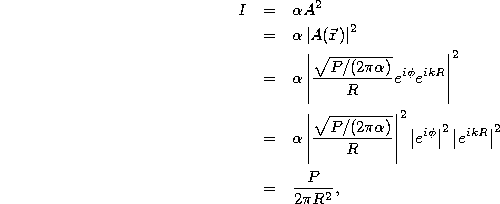where we have substituted Eq. 5 for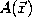and used the mathematical facts that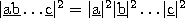for any product of complex numbers and that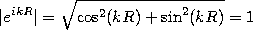.

The main lesson we learn from Eq. 6 is that for a single slit, the pattern on an observation screen at distance R from the slit is constant and uniform, independent of the angle of observation, as Figure 2 illustrates.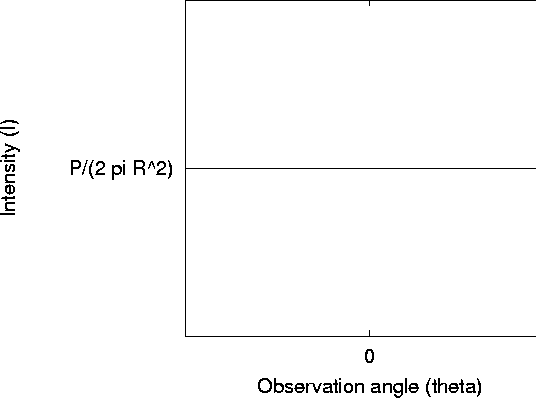Figure 2: Intensity as a function of observation angle for a single-slit is constant

Tomas Arias
Thu Sep 13 15:26:14 EDT 2001